# The Analytical Scheme on the Inertial Drag for Buoyancy-driven Nanofluid Flow Under Convective Thermal Surface with the Soret Effect

P.K. Pattnaik1
iD
, Subhajit Panda2, 3
iD
, S.R. Mishra4, *
iD
, Krushna K.P.N. Nayak4
1 Department of Mathematics, Odisha University of Technology and Research, Bhubaneswar, Odisha 751029, India
2 Department of Mathematics, Gandhi Institute for Technology, Bhubaneswar, Odisha, 752054, India
3 Department of Mathematics, National Institute of Technology Mizoram, Aizawl, 796012, India
4 Department of Mathematics, ITER, Siksha ‘O’ Anusandhan Deemed to be University, Bhubaneswar, Odisha 751030, India

#### Article Metrics

0
##### Total Statistics:

Full-Text HTML Views: 327
Abstract HTML Views: 115
##### Unique Statistics:

Full-Text HTML Views: 157
Abstract HTML Views: 78open-access license: This is an open access article distributed under the terms of the Creative Commons Attribution 4.0 International Public License (CC-BY 4.0), a copy of which is available at: https://creativecommons.org/licenses/by/4.0/legalcode. This license permits unrestricted use, distribution, and reproduction in any medium, provided the original author and source are credited.

* Address correspondence to this author at the Department of Mathematics, ITER, Siksha ‘O’ Anusandhan Deemed to be University, Bhubaneswar, Odisha 751030, India; E-mail: satyaranjan_mshr@yahoo.co.in

## Abstract

### Introduction:

The two-dimensional mixed convection of nanofluid over a vertical expanding surface is analysed in the current discussion. The expanding surface is embedded in a permeable medium.

### Methods:

In advance, Darcy Forchheimer inertial drag is considered along with the influence of Brownian and thermophoresis, which enriches the study. The novelty of the study is due to the mass concentration along with the role of volume concentration in the flow phenomena. The proposed model is designed in association with a characterizing parameter, which is attained by the use of appropriate similarity conversion. Further, the system of first-order differential equations is resolved by employing a shooting-based numerical method, in particular, the Runge-Kutta fourth-order technique.

### Results:

The simulated results for the said parameters and their behaviour are deployed through graphs and in tabular form.

### Conclusion:

The physical description of each parameter is deliberated briefly. Finally, the important outcomes of the proposed study reported a remarkable hike in the temperature profile that is observed for the enhanced thermophoresis and Brownian motion. Further, the shear rate also increases for the enhanced mixed convection parameter.

Keywords: Nanofluid, Mixed convection, Darcy-Forchheimer, Thermal radiation, Volume concentration, Mass concentration.

## 1. INTRODUCTION

Soret effect or thermophoresis is nothing but the phenomenon of showing numerous responses to the force of a temperature gradient by different particles of a mixture. It has different applications in practical situations like the separation or mixing of particles and differentiation of the impure ions in a chemical process, and it is also used as commercial precipitators. This phenomenon of gaseous mixtures was first introduced by John Tyndall, and later it was described by John Strutt. Similarly, mixed convection is a process that occurs when natural convection and forced convections combine together in a mechanism to transfer heat. In some cases where forced convection is not efficient, at that time, mixed convection gives better results. The process is used in various fields, such as in nuclear reactor technology, some electronic cooling processes, etc. Again, the convective boundary condition is also known as the Newton boundary condition. Many researchers have given many results on mixed convection flow, the Soret effect and convective boundary conditions.

Khan et al.  addressed the dual diffusion heat mechanism of nanomaterials under the action of thermal radiation and temperature absorption/production. In order to discover the analytical expressions, the Homotopy analysis approach is enforced. Khan et al.  studied micropolar nanofluid in three dimensions along with the impact of thermal radiation, activation energy and magnetic effects. Song et al.  identified the features of activation energy along with the thermal radiation in micropolar nanofluid with motile microorganisms through a stretched sheet. Waqas et al.  analysed the bio-convection transport of micropolar-based nanomaterials and gyrotactic motile microorganisms’ consequence with thermal radiation and activation energy past a needle moving in parallel flow. Pattnaik et al.  explored the impression of the shape of nanoparticles on the conducting nano-structured fluids via a porous matrix. Afzal et al.  studied the nanoparticles in the stream of general micropolar fluid along with Carreau fluid across a flat surface. El-Dawy and Gorla  examined a steady laminar flow of microstructured nanofluid via an extended and contracting wedge with the effect of heat production or preoccupation and an induced magnetized field across a wedge surface.

Salmi et al.  inspected the impression of motile organisms in microstructure fluid in the context of simultaneous thermal and mass transmission along with thermal diffusion. Baag et al.  explored the behaviour of radiant heat on a moment flow of MHD microstructure fluid towards upward overextended sheet with the collaboration of a transverse magnetic field along with the Soret effect and convective conditions. Khan et al.  analysed the buoyancy impact on moment flow caused by a hybridized microstructure nanofluid across a porous plate. Hussain and Xu  reported a non-Newtonian magnetohydrodynamic (MHD) micropolar fluid in which the nanofluid theory is implemented to discuss the thermophoresis and the Brownian motion phenomenon along with bioconvection and buoyancy forces. Barik et al.  investigated the circulation of hydromagnetic nanostructured fluid, including the consequences of a slippery situation responding to an elastic interface. Additionally, Mishra et al.  have published the influence of nonlinearly radiated via an elongating interface employing mutual diffusion as well as magnetic heat flux efficiency. The Adomian decomposition method was used to model thermal energy transmission in the nanofluid over a semi-infinite straight sheet by a porous media analyzed by Mishra et al. . Pattnaik et al.  implemented the technique developed by Runge-Kutta with a shooting approach to examine two-dimensional magnetohydrodynamic (MHD) flow in a nano-structured fluid together with the implications of different physical variables. In a recent study, Krzywanski  explores improved heat transfer performance in an industrial circulating fluidized bed combustion (CFBC) system by using fuzzy logic-based techniques. In another work, Krzywanski et al.  investigated an AI-based approach to predict heat and mass transfer in fluidized beds, offering potential insights into cooling and desalination processes.

The earlier investigations presented through the aforesaid literature show that not much work on the case of assisting/opposing has been conducted for the flow of nanofluid. Therefore, the mixed convection effect with assisting/opposing is deployed for the flow of nano-structured fluid with the implication of Darcy-Forchheimer inertial drag along with thermal radiation. The objective of the study is to develop an analytical framework for understanding and predicting the inertial drag in buoyancy-driven nanofluid flow. The study specifically focuses on nanofluids subjected to convective thermal surface conditions and considers the influence of the Soret effect. By establishing an analytical scheme, the researchers aim to provide insights into the complex interplay between fluid flow, heat transfer, and nanoparticle migration in such systems. The developed framework can contribute to the design and optimization of nanofluid-based technologies with enhanced thermal performance.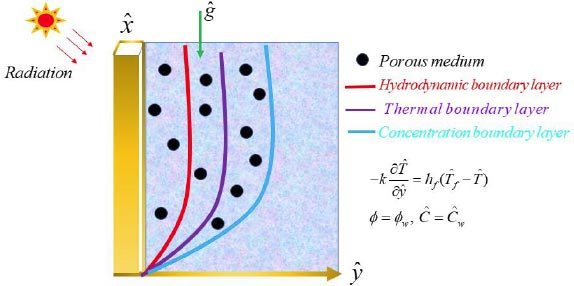Fig. (1). Physical fluid model.

## 2. MATERIAL AND METHOD

A two-dimensional coordinate system is contemplated in which the y-axis is perpendicular to the x-axis and flows towards the horizontal direction. A continuous mixed convective heat and mass transmission are considered via a vertical and flat sheet that is implanted in a nano-structured fluid consuming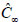(concentration)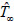(temperature) and φ (nanoparticle volume fraction) in the ambient medium. Also, a free stream with steady velocity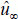passes via a flat plate is considered (Fig. 1).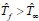agreeing to assis flow i.e., heated surface and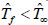agreeing to oppose flow i.e., cooled interface.

• The fluid viscosity and the volume of the nanoparticles on the wall are reserved to be fixed and are specified by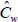and Øw correspondingly.

The leading equations derived by using the Oberbeck-Boussinesq approximation, using the conventional boundary layer approximation and removing pressure are given by: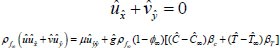(1)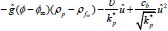(2)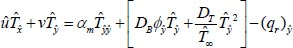(3)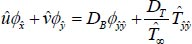(4)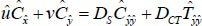(5)

As well as boundary restrictions: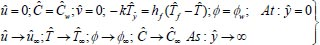(6)

Where ѱ function is given by: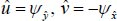(7)

And let us take the following non-dimensional transformations: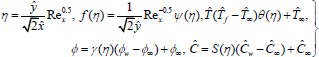(8)

Now substituting equation (7) from equations (2) to (5) and then using the above transformations, we have;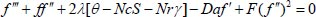(9)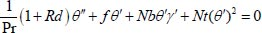(10)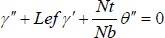(11)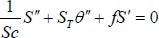(12)

Here, the prime notations indicate the differentiation with respect to η. Also, the conditions in (6) in terms of ƒ, θ, γ, S reduce to: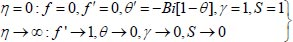(13)

The interesting and important aspects of the shear rate Cƒ and heat transfer rate Nu are presented as,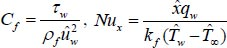(14)

Here, τw is skin friction, qw is heat flux at the surface is: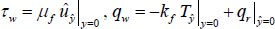(15)

yields to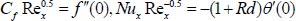(16)

Here,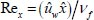, Reynold’s number is presented locally.

## 3. NUMERICAL METHODOLOGY

The standard modelled equations articulated in equations (9-12) are nonlinear ODEs. Here, we have applied a semi-analytical method to solve the equations known as the Adomian Decomposition Method.

First, we introduce the operators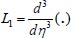and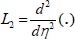with inverse operators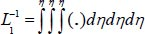and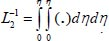. Thus, by applying ADM, the equations (9-12) can be written as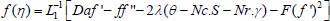(17)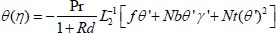(18)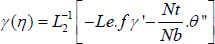(19)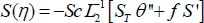(20)

ƒ(η), g(η), θ(η) and ϕ(η) can be expressed in the power series form stated as: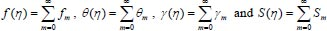(21)

For the rest terms of the equations (17-20), take the power series expansions as: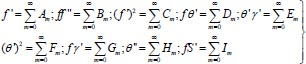(22)

From equation (13) and the assumed initial conditions are: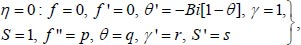(23)

where the values of p, q, r, s are to be resolute.

Applying these conditions, the initial and succeeding order solutions are articulated as: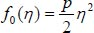(24)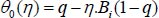(25)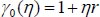(26)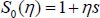(27)

And: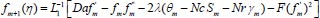(28)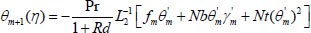(29)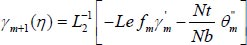(30)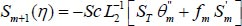(31)

Putting the values of m = 0,1.... one can obtain the solution of the governing equations as: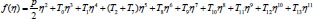(32)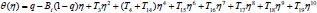(33)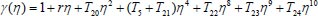(34)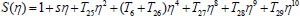(35)

However, for the sake of brevity,the values of T'is are omitted.

## 4. RESULTS AND DISCUSSION

The subject presents the buoyancy-driven nanofluid flow toward an extending vertical surface entrenched with a porous media. The Darcy-Forchheimer inertial drag with the effect of thermophoresis and Brownian motion enriches the study. One of the vital aspects of the present study is the inclusion of the volume concentration. The designed model for the governing modelled equations is solved by employing a semi-analytical technique followed by suitable similarity transformations that are helpful for making non-dimensional for of flow phenomena along with the surface conditions. In particular, the Adomian decomposition method is deployed for the solution of the system. The behaviour of the particular constraints is exhibited and displayed graphically. However, the simulations for CƒRex0.5 and NuRex0.5 are deployed via the tabular form. The computation involves the fixed values of the constraints, such as Da = 0.2, F = 1, Bi = 0.1, λ = 0.2, Nt = 0.3, Nb = 0.2, Nc = 0.1, Rd = 0.3, Pr = 2, Le = 1, Sc = 0.1, St = 0.1 where the standard variation of each parameter is displayed in the corresponding figures.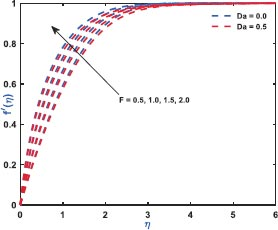Fig. (2). Convergence of F on ƒ'(η).

### 4.1. Description of Parametric Behaviour

Fig. (2) exhibits the role of inertial drag due to the existence of a porous matrix on the ƒ'(η). It is the force that pushes the fluid away from the moving body that differs from viscous force. The Figure shows the variation with respect to the appearance (Da = 0.5)/non-appearance (Da = 0) of the Darcy parameter. It clarifies that enhanced drag overshoots the velocity distribution irrespective to the variation of Darcy parameter for which the thickness of the bounding surface retards significantly. Fig. (3) reveals the noteworthy features of the mixed convection parameter on the fluid velocity. The impact is due to the variation of the thermal Grashof number i.e., buoyancy factor. Buoyancy is an upward force that is observed owing to the pressure differential between the fluid layers. The top layer pressure is dominated by the bottom and the fluid at bottom moves away for which the fluid velocity enhances throughout. Fig. (4) signifies the role of regular buoyancy parameter affecting the fluid velocity for the variation of Darcy parameter. It is defined as the ratio of the concentration difference with the temperature differential between the wall and the boundary. The increasing buoyancy of the temperature difference retards so it affects the fluid velocity and therefore, the fluid velocity also decreases significantly. Fig. (5) reflects the influence of nanofluid buoyancy parameter on the fluid velocity. It is defined as the ratio of the concentration difference with respect to the temperature difference. A similar effect is rendered as previous since, the enhanced buoyancy is due to the retardation in the temperature difference vis-à-vis the concentration increases, therefore the fluid velocity attenuates gradually. The presence of permeability also attenuates ƒ'(η) greatly comparing to the impermeability of the medium. Fig. (6) elaborates the significance of Bi on the θ(η) in association with the involvements of permeability. The calculation of heat transport is conducted by the use of Bi. It is described as the thermal resistance ratio for the conduction inside the body with respect to the resistance for the convection at the upper surface. The numerical value of the Biot number less than unity shows the thermal profile is stable. The figure discloses that the enhanced Bi augments the θ(η) irrespective to the various Darcy numbers. However, increasing Darcy number enhances the temperature profile since the resistance is due to the permeability that stored near the sheet region that overshoots the profile. Fig. (7) characterizes the properties of Brownian motion θ(η). The two-layer difference within the domain demonstrates the behaviour of the Brownian motion for the effect of porosity. The increasing porosity enhances the fluid temperature, as discussed earlier, and the increasing Brownian motion also favors in augmenting the temperature profiles. Fig. (8) illustrates the role of thermophoresis that affects θ(η). It occurs due to the diffusion of the thermal gradient in γ(η) and the augmentation in the thermophoresis parameter, and the nanofluid temperature rises up for which it enhances the profile asymptotically. However, the observation reveals that the impermeability of the surface provides greater control over temperature. Fig. (9) portrays the significance of the radiating heat approaching to a linear radiative model on the fluid temperature profiles. It is used as a measure for the amount of emitted electromagnetic radiation from the fluid elements. This electromagnetic radiation is further transformed into thermal radiation. The result shows a dual behaviour within the domain. It can be seen that near the sheet region the profile was retard but with increasing domain, it shows opposite behaviour on the profile. Fig. (10) characterizes the role of the Lewis number on the profiles of volume fraction. The occurrence of Lewis number is owing to the existence of the Brownian diffusion coefficients. It is expressed as the ratio of the thermal diffusion and the Brownian diffusion. Therefore, enhancing Lewis number occurs for the decreasing Brownian diffusion and it helps to retard the volume concentration profile. Figs. (11 and 12) portray the role of the Brownian factor along with thermophoresis factor on the volume faction profiles. The behaviour shows the variation of permeability on the profiles. Here, the volume concentration attenuates for the increasing Brownian motion but the profile behaves in opposite direction for the increasing thermophoresis. The comparative analysis exhibited that the situation of permeability overrides the situation of impermeability of the medium. Fig. (13) illustrates the behaviour of the Sc affecting the nanofluid concentration distribution with the variation of porous matrix. The existence of the Schmidt number is due to the occurrence of heavier species considered in the flow phenomena. The expression of Schmidt number describes the relationship between the kinematic viscosity and the solutal diffusivity. The profile attenuates with the increasing Schmidt number therefore; the heavier species has a greater control in reducing fluid concentration. Further, Fig. (14) elaborates the experience of the Soret number on the fluid concentration. When the fluid temperature diffuses in the concentration distribution, the fact is termed as Soret. The result depicted that increasing Soret increases the concentration distribution asymptotically. Moreover, in both the figures, it is clearly visible that the flow via impermeable medium favors in controlling the fluid concentration, whereas the flow through permeable surface overshoots the profile significantly.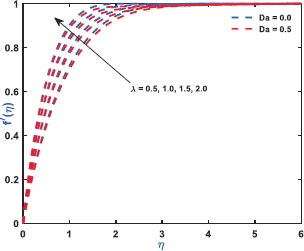Fig. (3). Convergence of λ on ƒ'(η).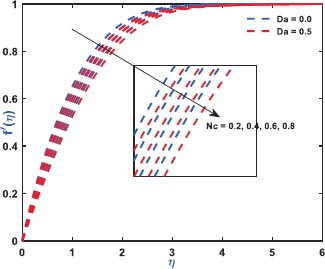Fig. (4). Convergence of Nc on ƒ'(η).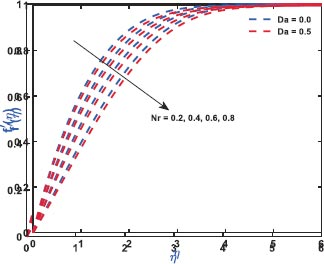Fig. (5). Convergence of Nr on ƒ'(η).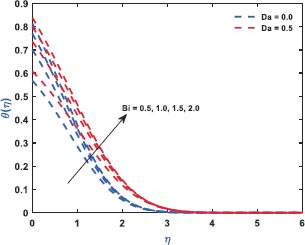Fig. (6). Convergence of Bi on θ(η).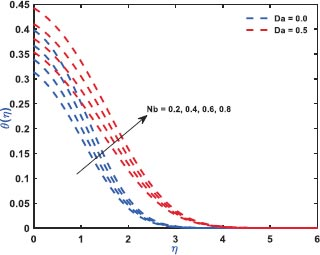Fig. (7). Convergence of Nb on θ(η).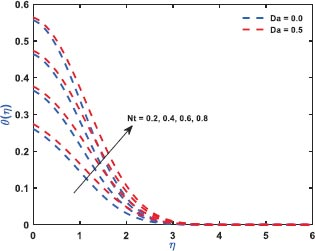Fig. (8). Convergence of Nt on θ(η).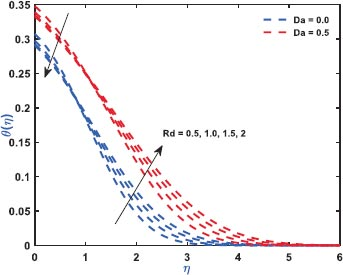Fig. (9). Convergence of Rd on θ(η).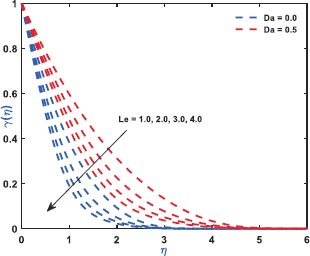Fig. (10). Convergence of Le on γ(η).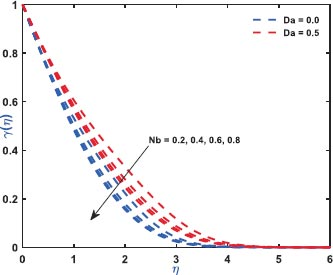Fig. (11). Convergence of Nb on γ(η).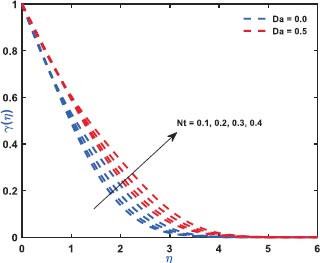Fig. (12). Convergence of Nt on γ(η).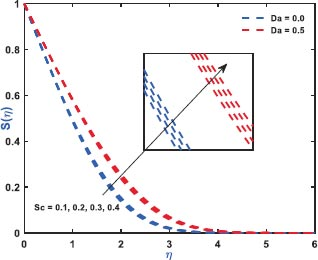Fig. (13). Convergence of Sc on γ(η).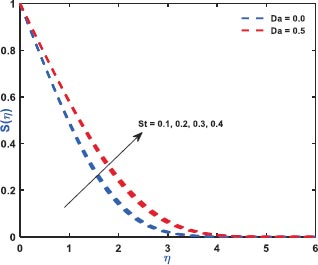Fig. (14). Convergence of St on γ(η).

Finally, it is important to discuss the numerical outcomes for the simulated values of the rate coefficients such as CƒRex0.5 and NuRex-0.5. Table 1 displays the variation of diversified constraints on the profiles of shear rate. The observation discloses that for the augmentation in the regular buoyancy, the nanofluid buoyancy ratio and the Darcy number due to the permeability of the medium retards the shear rate coefficients, whereas the mixed convection parameter and the Darcy-Forchheimer inertial drag overshoots the profiles of shear rate significantly. Further, Table 2 depicts the behaviour of characteristic factors on the heat transfer rate coefficients to see the performance of the Nusselt number.

Table 1. Numerical Computation of CƒRex0.5.
 λ Nc Nr Da F CƒRex0.5 0.1 0.2 0.9 0.8 0.8 0.4232 0.2 - - - - 0.4902 0.3 - - - - 0.5545 0.1 0.2 - - - 0.4417 - 0.4 - - - 0.3431 - 0.6 - - - 0.2411 - 0.2 0.3 - - 0.3927 - - 0.5 - - 0.2926 - - 0.7 - - 0.1882 - - 0.9 0.3 - 0.3471 - - - 0.5 - 0.2068 - - - 0.7 - 0.1551 - - - 0.8 0.2 0.3562 - - - - 0.4 0.3841 - - - - 0.6 0.4154 - - - - 0.8 0.4507
Table 2. Numerical Calculation of Nu Rex-0.5.
 Pr Nb Nt Rd Nu Rex-0.5 0.71 0.1 0.5 0.5 0.0884 1 - - - 0.0881 2 - - - 0.0851 0.71 0.1 - - 0.087 - 0.2 - - 0.0851 - 0.3 - - 0.083 - 0.1 0.2 - 0.0917 - - 0.3 - 0.0851 - - 0.5 - 0.072 - - 0.2 0.5 0.0992 - - - 1 0.1344 - - - 1.5 0.1693 - - - 2 0.2038

## CONCLUSION

The mixed convection of nanofluid flow for the influence of Brownian and thermophoresis factors in association with the radiative heat flux is analysed in the existing discussion. The insertion of Darcy-Forchheimer inertial drag along with the volume fraction enhances the flow properties as well. The shooting-based numerical method, such as the traditional Runge-Kutta method, is used for the solution of the transformed model for various factors. Further, the significant outcomes for these parameters are described, and a few important characteristics are laid down here.

• Irrespective of the attendance/ non-attendance of the permeability of the medium the inertial drag by reason of the coefficient enhances inertial drag force, i.e., Darcy-Forchheimer the fluid velocity, and this causes the smooth retardation in the surface thickness.

• The mixed convective factor intended for the concurrence of the thermal buoyancy factor exceeds the fluid velocity, but a significant attenuation is observed for the regular and nanofluid buoyancy ratio parameters.

• Thermophoresis, along with the Brownian motion factor in occurrence Bi due to the heat flux boundary restrictions, favors in enhancing the fluid temperature throughout, but the flow along impermeable medium restricts it.

• The mutual consequence of thermophoresis as well as the Brownian factor, also shows a dual characteristic on the volume fraction profiles since the profile retards with increasing Brownian motion, but an opposite trend is observed for the thermophoresis.

• The retardation in the Brownian diffusion helps in enhancing the Lewis number, and this is the parameter that favors controlling the volume concentration for its increasing behaviour.

• The mixed convection parameter for the flow over a permeable medium augments the shear rate, but the regular, as well as nanofluid buoyancy ratio parameter, retards it significantly.

Finally, the present study has several practical applications, such as the findings of this study can be applied to improve the efficiency of energy systems that utilize nanofluids. By understanding the inertial drag and convective thermal surface effects, engineers can optimize the design of heat exchangers and thermal management systems for enhanced energy transfer. However, the behavior of nanofluids under convective thermal surfaces can be useful in the development of advanced cooling technologies. This knowledge can aid in designing efficient cooling systems for electronic devices, power plants, and other industrial processes. It has implications in biomedical engineering, particularly in the field of hyperthermia treatment. By understanding the inertial drag and the Soret effect, researchers can optimize the delivery of heat to target areas in tumor tissues, leading to improved therapeutic outcomes. However, one can extend their work by considering the nonlinear thermal radiation with the multiple slip effect, and those have several applications, as mentioned earlier. Also, instead of using the same methodology, authors can use the analytical methods likely, differential transform with Pede approximation, Variation parameter method, Homotopy perturbation method, etc. As a challenging area, the statistical approach along with Artificial Neural Network, can be employed for the optimized heat transfer rate.

### NOMENCLATURE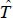Temperature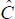Solutal concentration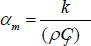Thermal diffusivity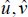Components of velocity towards and axes

ρƒ∞ Density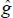Gravitational Acceleration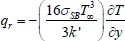Thermal radiation

σSB Stefan-Boltzmann constant

k Thermal conductivityKinematic viscosity coefficient

k' Mean absorption coefficient

ρ Density

βT Thermal expansion coefficient for volume

βc Solutal expansion coefficient for volume

kP* Permeability

ρP Density of the nano particles

DCT Soret type diffusivity

(ρC)P Effective heat capacity

DT Thermophoretic diffusion coefficient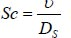Schmidt number

Ds Solutal diffusivity

µ Viscosity

DB Brownian diffusion coefficient

hƒ Convective heat transfer coefficient

ѱ Stream function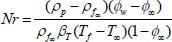Nanofluid buoyancy ratio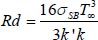Prandtl number

(ρC)ƒ The heat capacity of the fluid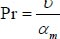Lewis number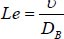Consistent buoyancy ratio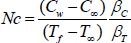The local Grashof number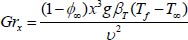Brownian motion factor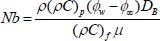Soret number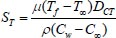Biot number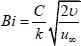Thermophoresis parameter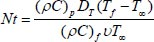Darcy Number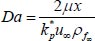Drag Force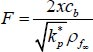Mixed convection parameterRadiation Parameter

Not applicable.

Not applicable.

None.

### CONFLICT OF INTEREST

Dr. S.R. Mishra is the Editorial Board Member of The Open Chemical Engineering Journal.

Declared none.